# ELU activation: A comprehensive analysis

Exponential Linear Unit (ELU), proposed by Djork-Arné in 2015, is a variant of the so-called ReLU nonlinearity. Through various experiments, ELU is accepted by many researchers as a good successor of the original version (ReLU).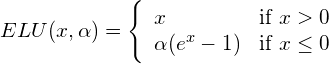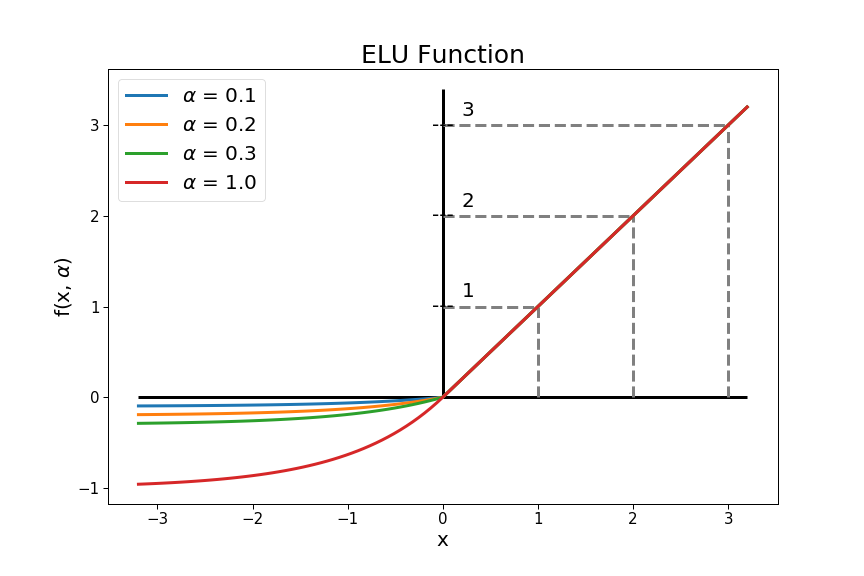ELU Function for different . Note that the value is pre-defined by the user, i.e. it is not learned by the network.

While ELU’s output may not be smaller than or equal to –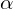, the upper bound is clearly undefined (it goes to infinity). Hence, ELU is a non-saturated nonlinearity.

In practice,is often set to be 1.0 or in the range [0.1, 0.3].

The derivative of ELU is given by: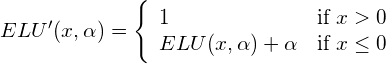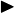The dying ReLU problem no longer exists, as both negative and positive inputs are transformed to non-0 outputs.Outputs can be either positive or negative. Studies showed that functions with 0-centered outputs help networks train faster. Although ELU’s outputs are not distributed around 0, the fact that it does produce negative values makes it be preferred in this sense compared to ReLU. In practice, it seems that networks with ELU converge more quickly than with ReLU, even though the exponential computation in ELU (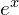) requires longer processing time.ELU is not piece-wise linear, this makes it model the non-linearity better.The plateau in the negative region helps in maintaining robustness and stability.is fixed, not learned.It still suffers from Gradient Exploding and Gradient Vanishing Problem, thus the help of normalization methods may remain necessary sometimes (e.g. this paper). SELU, which was built from ELU with an innate ability to self-normalize, was introduced to address this problem.It is not 0-centered. Although ELU does produce negative outputs, the fact that it is not 0-centered makes it seemingly sub-optimal. Another nonlinearity called Parametric ELU is introduced to mitigate this issue.No sparsity. ReLU outputs 0 for negative inputs, which is bad in some senses but also good in some others, as elaborated in this post.

## Performance Comparison

### Experiment from ELU’s authors

#### Experiment 1:

Objective: To compare the performance of ELU with ReLU and Leaky ReLU on a simple classification task.

Experimental Design:

• Dataset: MNIST
• Network: 8 hidden layers of 128 neurons each. The networks are trained by stochastic gradient descent (SGD) with a learning rate of 0.1 and a mini-batch size of 64.
• ELU with= 1
• Leaky ReLU with= 0.1

Result: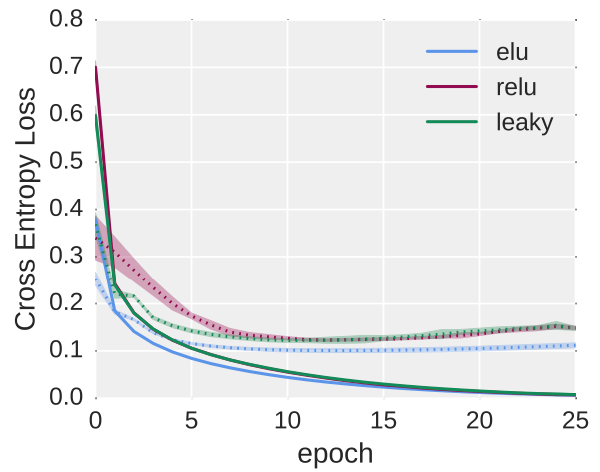Networks’ loss using the 3 types of nonlinearity. The straight lines represent training errors while the dotted lines show testing errors.

Verdict: While ReLU and Leaky ReLU had similar behaviors after 10 epochs of training with the loss around 0.15, ELU expressed a stronger power that nearly converged after 5 epochs with only 0.1 cross-entropy loss.

#### Experiment 2:

Objective: To compare the performance of ELU versus ReLU and Leaky ReLU on unsupervised learning.

Experimental Design:

• Dataset: MNIST
• Network: a deep autoencoder whose encoder part consists of 4 fully connected hidden layers with sizes 1000, 500, 250, and 30, respectively, while the decoder part is totally symmetrical. There are different fixed learning rate in use. The networks are trained by stochastic gradient descent with mini-batch sizes of 64.
• ELU with= 1
• Leaky ReLU with= 0.1

Result: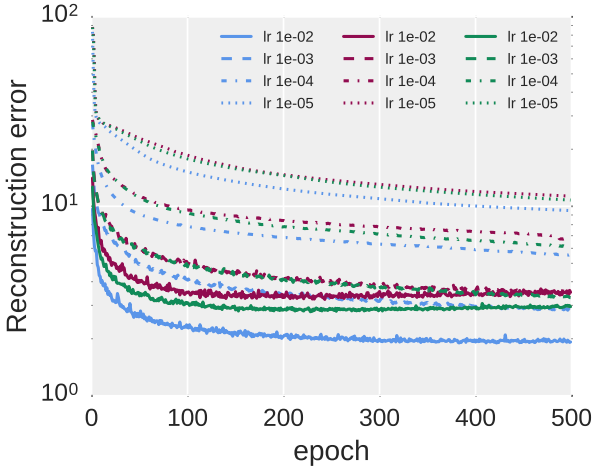Network recovering errors with different types of nonlinearity. All the errors are measured on the testing set. The blue, red and green colors represent ELU, ReLU and Leaky ReLU, respectively.

Verdict: This result implies the superiority of ELU over the other 2 activations while its error rates are clearly the lowest for every choice of learning rate.

#### Experiment 3:

Objective: To compare the performance of ELU versus ReLU, Leaky ReLU and SReLU on a more complex supervised learning task.

Experimental Design:

• Dataset: CIFAR-100, which contains color images in 100 classes, 50k train, and 10k test. The images were also preprocessed with global contrast normalization, ZCA whitening, and 4 0-pixels padded at all borders.
• Network: a CNN with 11 convolutional layers in stacks. A 2×2 max-pooling with a stride of 2 was applied after each stack. Dropout and L2-weight decay regularization are used. The learning rate is decreased in iterations.
• The networks are trained with and without BatchNorm.

(More details about the configuration can be found in the paper here.)

Result:

Without BatchNorm: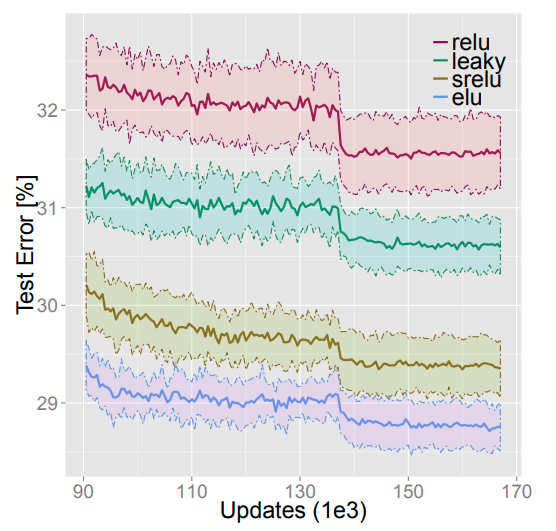Networks’ test errors (and their standard deviations) on CIFAR-100 dataset using different nonlinearities. BatchNorm is not used.

With BatchNorm: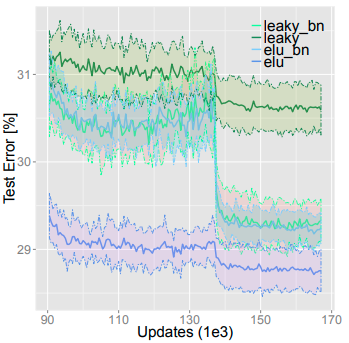Performance comparison between ELU and each of the 3 other nonlinearities.

Verdict: It is shown that ELU outperforms the remaining activations, even when they are helped by BatchNorm. Furthermore, there is some evidence that adding BatchNorm does not enhance a network with ELU but may even make its error rate higher.

#### Experiment 4:

Objective: To compare the performance of a CNN network with ELU versus other renowned CNN architectures.

Experimental Design:

• Dataset: The CIFAR-10 and CIFAR-100 datasets. Data preprocessing is similar to the previous experiment.
• Network: The CNN with ELU is structured as: there are 18 convolutional layers. The dropout rate, Max-pooling, L2-weight decay, momentum term are similar to the previous experiment. The initial learning rate is set to 0.01 and decreased by a factor of 10 after each 35k iterations. The mini-batch size is 100.

(More details about the configuration can be found in the paper here.)

Result: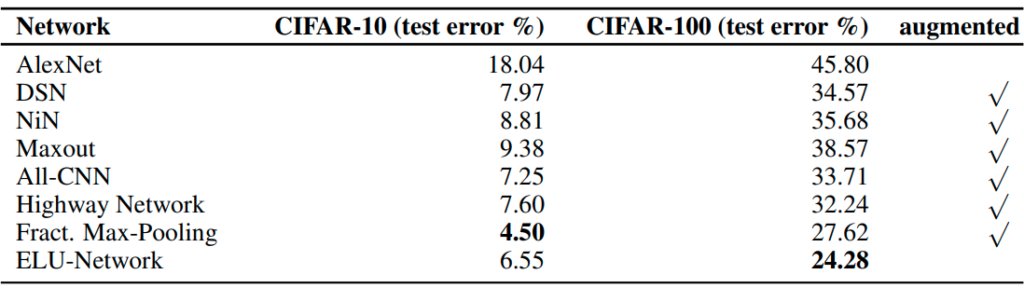The result of an experiment that compares a network with ELU vs other known architectures.

Verdict: It is shown that the network with ELU gave the lowest error rate for CIFAR-100. Furthermore, it is also ranked second on CIFAR-10.

#### Experiment 5:

Objective: To compare ELU and ReLU on the ImageNet dataset.

Experimental Design:

• Dataset: the 1000-class ImageNet. Images are resized to 256×256 pixels and per-pixel mean subtracted. Training is performed on 224×224 random crops with random horizontal flipping. No augmentation during training.
• Network: The network has 15 convolutional layers. 2×2 max-pooling with a stride of 2 is applied after each stack. Spatial pyramid pooling with 3 levels is added 3 levels before the first fully-connected (FC) layer. L2-weight decay term to 0.0005 is used. The dropout rate is 50% for 2 penultimate FC layers.

Result: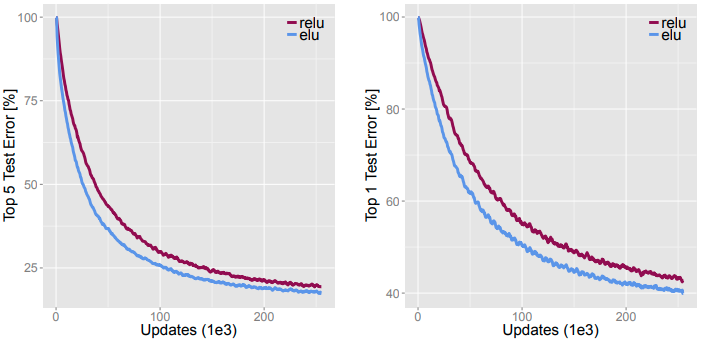The comparison between ELU and ReLU on the ImageNet dataset. On the left is Top-5 Test error while on the right is Top-1 Test error.

Verdict: This result shows that the network with ELU reduces the error rate faster than with ReLU in terms of the number of iterations. To reach a 20% Top-5 error rate, ELU needs only 160k iterations, this number is 200k iterations for ReLU.

Note that the speed of using ELU is slower by 5% compared with using ReLU.

### Experiments from others

#### Experiment 1:

Objective: To compare the performance of the original ResNet (which uses a combination of ReLU + BatchNorm) and the same network but changed to use ELU instead.

Experimental design:

• Dataset: CIFAR-10
• Network: The original ResNet (with various depths) is used as the benchmark. The new ResNet (that with ELU) is changed a bit in structure, as showed below.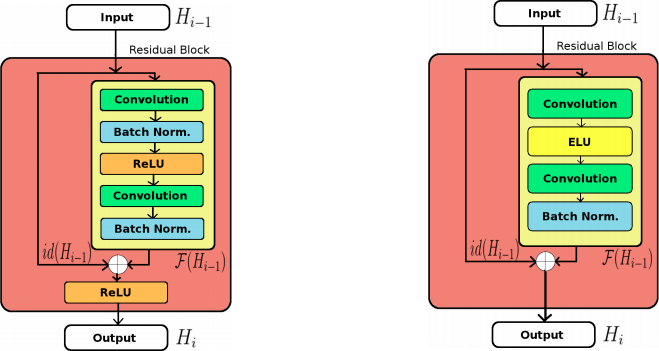On the left is the i-th Residual Block in the original Residual Network. On the right is the tweaked version, which changed from using ReLU to ELU and modified the internal organs a little bit.

Result:

The 2 architectures are trained with different depths.Comparison of testing error between the original and modified ResNet on various numbers of layers.

Verdict: The new ResNet with ELU gives higher performance regardless of the depth.

#### Experiment 2:

Objective: To compare the new network architecture (the modified ResNet using ELU) with other state-of-the-art methods.

Experimental design:

• Dataset: CIFAR10 and CIFAR-100
• Network: The same modification is applied to ResNet as stated in the experiment above.

Result:

Verdict: The modified ResNet with ELU shows the best performance.

#### Experiment 3:

Objective: The original purpose of this experiment is to highlight the effectiveness of Edge of Chaos (EOC), which is a specific choice of hyperparameters as described in Schoenholz, 2017. However, we can adapt the result to compare the time to convergence of networks using ELU, ReLU and Tanh nonlinearities.

Experimental design:

• Dataset: MNIST
• Network: The networks have their depth of 200 and width of 300. Networks are trained with RMSProp. The mini-batch size of 64 is used. The learning rate is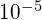.

(More details about the configuration can be found in the paper here.)

Result: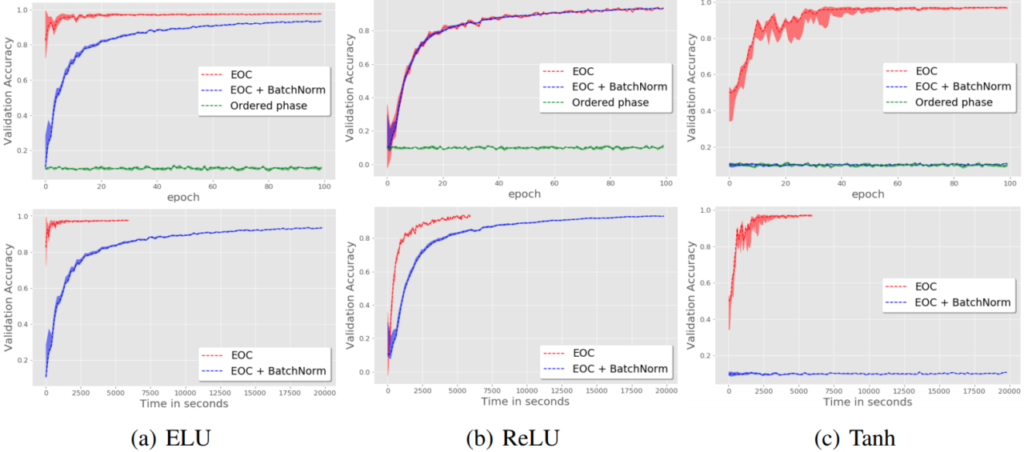The experimental result on the MNIST dataset. The upper figures show the accuracies with respect to the epochs while the lower figures show the accuracies with respect to time.

Verdict: In terms of speed, ELU outperforms ReLU and Tanh in this experiment, given that it converges after sufficiently less time and epochs than the other 2 activations.

#### Experiment 4:

Source: sonamsingh19.github.io

Objective: To compare the performances of ReLU and ELU activation functions for long dependencies.

Experimental design:

• Dataset: MNIST. The data is fetched sequentially (i.e. one pixel at a time).
• Network: an RNN with initialization using the identity matrix (IRNN).

Result:

Verdict: ELU reaches an accuracy of around 96% after 15 epochs while ReLU struggles below 85%.

## Conclusion

In this article, we discussed the ELU activation function for Deep Learning. Compared to the canonical ReLU, ELU is a bit more involved in the computation, however, with its various advantages, in practice, networks using ELU is not significantly slower than using ReLU (in fact, some experiments indicate that using ELU may even boost the speed for a large amount). Furthermore, there is evidence that ELU outperforms many other activations in terms of accuracy and error rate. Henceforth, many researchers have assumed using ELU as an enhanced version to replacing ReLU as their default nonlinearity.

References:

• The original paper of ELU by Djork-Arné: link
• Deep Residual Networks with Exponential Linear Unit by Shah et al.: link
• On the Impact of the Activation Function on Deep Neural Networks Training by Hayou et al.: link
• ML Low hanging Fruit: If you are using RNN for long dependencies: Try ELU: link
• A Simple Way to Initialize Recurrent Networks of Rectified Linear Units by Quoc et al.: link
• Deep Information Propagation by Schoenholz et al.: link